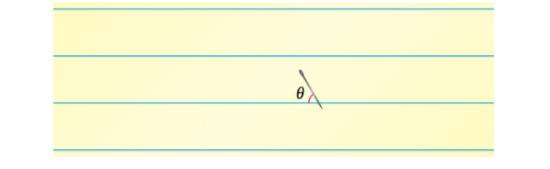Chapter 4.4, Problem 61E

Chapter
Section
Textbook Problem

Buffon's Needle Experiment A horizontal plane is ruled with parallel lines 2 inches apart. A two-inch needle is tossed randomly onto the plane. The probability that the needle will touch a line is P = 2 π ∫ 0 π / 2 sin θ d θ where θ is the acute angle between the needle and any one of the parallel lines. Find this probability.To determine

To calculate: The value of probability, P=2π0π2sinθdθ

Explanation

Given:

The needle falls according to the diagram

The probability that it will touch a line is P=2π0π2sinθdθ.

Formula used:

The probability can be solved by integrating the function within the limits according to formula

absinx=[cosx]ab

Still sussing out bartleby?

Check out a sample textbook solution.

See a sample solution

The Solution to Your Study Problems

Bartleby provides explanations to thousands of textbook problems written by our experts, many with advanced degrees!

Get Started

Find dy/dx by implicit differentiation. 13. x+y=x4+y4

Single Variable Calculus: Early Transcendentals, Volume I

Suppose the total cost function for a commodity is . 1. Find the marginal cost function.

Mathematical Applications for the Management, Life, and Social Sciences

Find each sum 634.719.45120.015_

Elementary Technical Mathematics